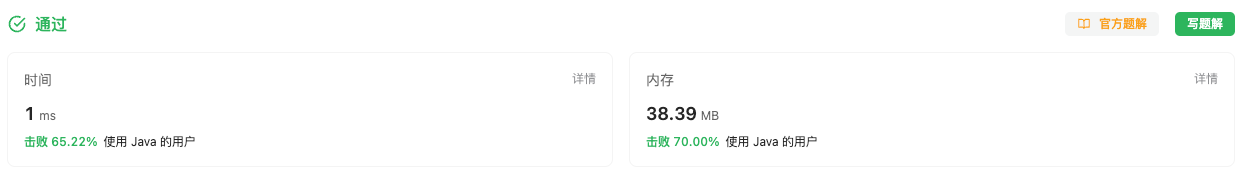#### 2605. 从两个数字数组里生成最小数字

###### 来源: 每日一题 2023.09.05

``````输入：nums1 = [4,1,3], nums2 = [5,7]

``````

``````输入：nums1 = [3,5,2,6], nums2 = [3,1,7]

``````

• `1 <= nums1.length, nums2.length <= 9`
• `1 <= nums1[i], nums2[i] <= 9`
• 每个数组中，元素 互不相同
``````class Solution {
public int minNumber(int[] nums1, int[] nums2) {

}
}
``````

#### 分析与题解

• 双指针

这个题目理解起来非常简单, 我们只需要先排序.

``````Arrays.sort(nums1);
Arrays.sort(nums2);
``````

然后使用双指针移动法进行移动, 如果双指针能找到对应的相等的数字直接返回.

``````int index1 = 0, index2 = 0;
while(index1 < nums1.length && index2 < nums2.length) {
if (nums1[index1] == nums2[index2]) {
return nums1[index1];
} else if (nums1[index1] < nums2[index2]) {
index1++;
} else {
index2++;
}
}
``````

如果查找不到, 那就找两个数组开头的数字组成一个较小的双位数返回即可.

``````return nums1 < nums2 ? (nums1 * 10 + nums2) : (nums2 * 10 + nums1);
``````

整体逻辑代码如下所示.

``````class Solution {
public int minNumber(int[] nums1, int[] nums2) {
Arrays.sort(nums1);
Arrays.sort(nums2);
int index1 = 0, index2 = 0;
while(index1 < nums1.length && index2 < nums2.length) {
if (nums1[index1] == nums2[index2]) {
return nums1[index1];
} else if (nums1[index1] < nums2[index2]) {
index1++;
} else {
index2++;
}
}
return nums1 < nums2 ? (nums1 * 10 + nums2) : (nums2 * 10 + nums1);
}
}
``````

复杂度分析:

• 时间复杂度: O(n), 一次遍历循环, 时间复杂度与较短的数组长度相关.
• 空间复杂度: O(1), 常量级别的空间复杂度.

结果如下所示.IT界无底坑洞栋主 欢迎加Q骚扰:676758285